## 基于原子体积场拉普拉斯算子对金属玻璃剪切转变区的预测 1)

* 中国科学院力学研究所, 北京 100190

† 中国科学院大学工程科学学院, 北京 100049

## PREDICTION OF SHEAR TRANSFORMATION ZONES IN METALLIC GLASSES BASED ON LAPLACIAN OF ATOMIC VOLUME 1)

Shi Ronghao*,, Xiao Pan,*,2), Yang Rong*

* Institute of Mechanics, Chinese Academy of Sciences, Beijing 100190, China

† School of Engineering Sciences, University of Chinese Academy of Sciences, Beijing 100049, China

 基金资助: 1)国家自然科学基金.  11790292国家自然科学基金.  11672298国家自然科学基金.  11432014中国科学院战略性先导科技专项(B类).  XDB22040501

Received: 2019-12-24   Accepted: 2020-02-15   Online: 2020-03-18Abstract

Shear transformation zone (STZ), as a basic characteristic unit of plastic events in metallic glasses (MGs), has been widely accepted by researchers, but the source of its origin and activation mechanism are still controversial. Deformation behaviours of Cu$_{64}$Zr$_{36}$ MGs under simple shear loadings are investigated using molecular simulation method in this paper. The results indicate that the activation locations of STZ are related to the initial configuration of MGs. Though the field of atomic volume and its gradient are a direct representation of the local atomic structural heterogeneity of MGs, they lack an obvious correlation to the regions of STZ activation. A new local structural parameter $\xi$ is proposed in this paper based on the initial configuration of MG to predict the potential regions of STZ. $\xi$ is the product of two factors: the Laplacian of atomic volume field (AVF) and the absolute difference between components of the gradient of AVF. Vectors of the AVF gradient present a distribution pattern of pointing inside if the Laplacian of AVF is negatively large, representing the localized soft regions in MGs. The absolute difference of AVF gradient components is used to select different patterns of the AVF gradient distribution. Furthermore, the relationship among structural parameter $\xi$, nonaffine displacement and shear localization is established, revealing that only certain patterns of AVF gradient distribution would lead to nonaffine displacements field strengthening shear localization, which is more likely to result in activation of STZs. The correlation analysis shows that the averaged spatial correlation index of $\xi$ and STZ is larger than 78%, so $\xi$ can be used as an effective parameter for predicting the activation regions of STZs in MGs. Moreover, the ideology of using Laplacian of local AVF in predicting potential STZ regions in MGs would bridge the analysis between atomic simulations of MGs, the mechanism of STZ activations and the traditional mechanical theory.

Keywords： metallic glasses ; shear transformation zones ; nonaffine displacements ; atomic volume ; molecular simulation

Shi Ronghao, Xiao Pan, Yang Rong. PREDICTION OF SHEAR TRANSFORMATION ZONES IN METALLIC GLASSES BASED ON LAPLACIAN OF ATOMIC VOLUME 1). Chinese Journal of Theoretical and Applied Mechanics[J], 2020, 52(2): 369-378 DOI:10.6052/0459-1879-19-369

## 1 计算模型和方法

3种金属玻璃样本均采用相同方法制备. 首先生成 Cu$_{64}$Zr$_{36}$ 面心立方晶体, 将该晶体在零压下加热到 2500 K 并弛豫 2 ns得到稳定的高温熔融液体. 然后以 10$^{13 }$ K/s 的降温速率将体系急冷至1 K并弛豫 100 ps 得到稳定的金属玻璃, 最后通过共轭梯度法进行能量极小化得到 0 K下Cu$_{64}$Zr$_{36}$ 的金属玻璃结构. 图2是所生成样品体系的径向分布函数(radial distribution function, RDF), 具有明显的玻璃态特征. 进而对样品进行非热准静态剪切加载(athermal quasi-static shear, AQS shear), 每一个加载步都对整个体系在 $x$ 方向上施加应变为 0.01%的简单剪切, 然后对体系进行能量极小化, 得到当前加载步的平衡状态用于构型和结果分析.

Table 1  Parameters of three sizes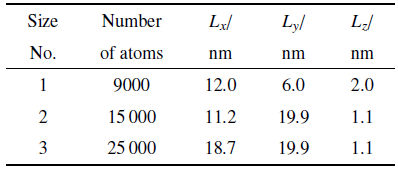### 图1Fig. 1   The configuration and dimensions of sample 1

### 图2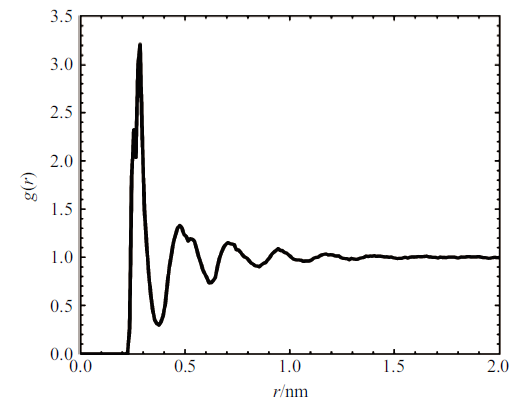Fig. 2   RDF of the prepared metallic glass sample

## 2 结果和讨论

### 图3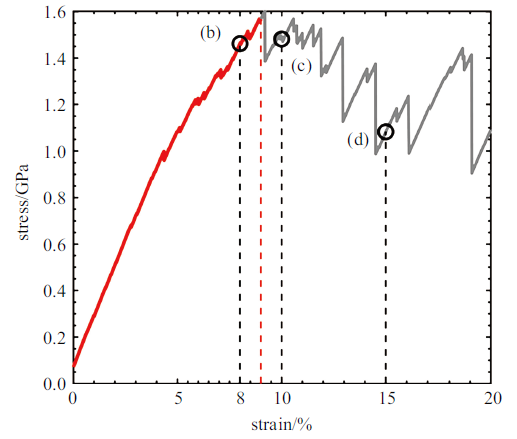Fig. 3   Stress strain curve of sample 1

### 图4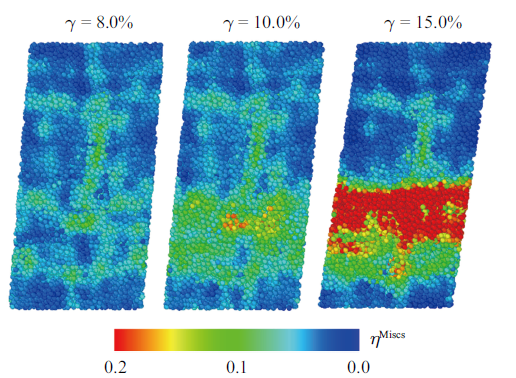Fig. 4   The distribution of atomic strain when $\gamma$ = 8.0%, 10.0% and 15.0%

### 图5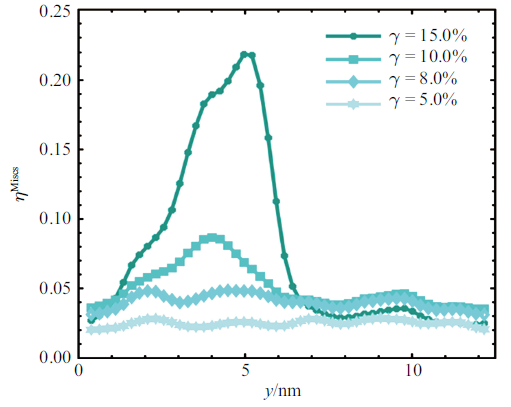Fig. 5   The distribution of averaged atomic strain along $y$ axis

\begin{equation} \label{eq1} \Delta \eta _i ^{\rm Mises}(\gamma )=\eta _i ^{\rm Mises}(\gamma )-\eta _i ^{\rm Mises}(\gamma -\Delta \gamma )>\Delta \eta _t ^{\rm Mises} \end{equation}

### 图6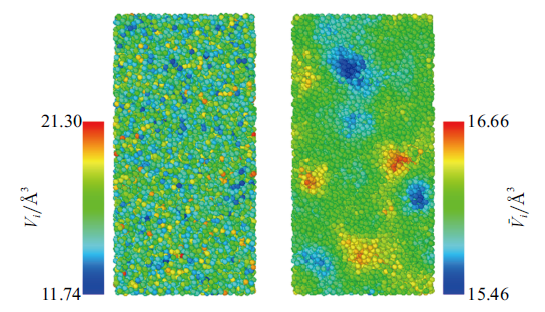Fig. 6   The distribution of $V_i$ (left) and $\overline V _i$ (right) in initial configuration

\begin{equation} \label{eq2} \nabla \overline V =\left( {\frac{\partial \overline V }{\partial x},\frac{\partial \overline V }{\partial y}} \right)=(\overline V _x ,\overline V _y ) \end{equation}

### 图7Fig. 7   The distribution of $\overline V _x$(left) , $\overline V _y$ (middle) in initial configuration and STZ atoms determined by Eq. (1)(right)

### 2.3 原子体积梯度与非仿射位移场的统计关系

\begin{equation} \label{eq3} \Delta r^\ast (\gamma )=r(\gamma )-r(\gamma -\Delta \gamma )-\Delta r^{\circ} \end{equation}

\begin{equation} \label{eq4} \varXi _{jk} =D_{ij} \left. {\frac{\mbox{d}r_i ^\ast }{\mbox{d}\varepsilon _k}} \right|_{\varepsilon _k =0} \end{equation}

\begin{equation} \label{eq5} r_j ^\ast =\frac{\varXi _j \varepsilon _j }{D_j } \end{equation}

\begin{equation} \label{eq6} \varXi _j =-\left. {\left( {\frac{\partial ^2U}{\partial r_j ^\ast \partial r_j ^{\circ}}} \right)} \right|_{r_j^\ast } \frac{\partial r_j ^{\circ}}{\partial \varepsilon _j }=a(k_{j+} -k_{j-} ) \end{equation}

\begin{equation} \label{eq7} r_j ^\ast =\varepsilon _j a\frac{k_{j+} -k_{j-} }{k_{j+} +k_{j-} }\approx\varepsilon _j a^2\frac{\partial K}{\overline K \partial x_j } \end{equation}

### 图8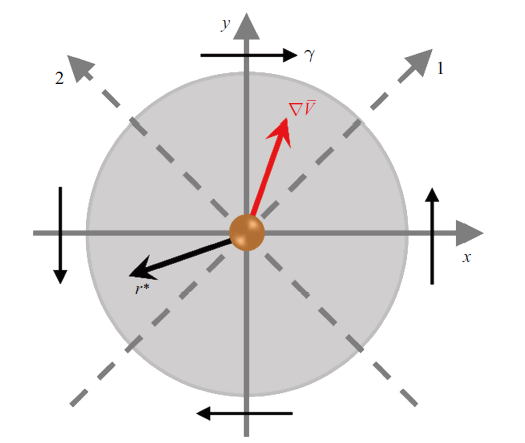Fig. 8   The relationship between nonaffine displacement and the heterogenous atomic volume

### 图9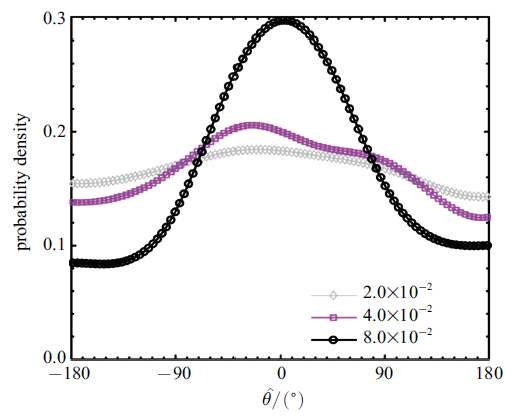Fig. 9   Distribution of angles between $-(\overline V _y ,\overline V _x )$ and $r^\ast$

### 2.4 原子体积梯度分布特征与剪切局部化

\begin{equation} \label{eq8} \nabla ^2\bar{{V}}=\nabla \cdot \left( {\frac{\partial \overline V }{\partial x},\frac{\partial \overline V }{\partial y}} \right)=\frac{\partial ^2\overline V }{\partial x^2}+\frac{\partial ^2\overline V }{\partial y^2} \end{equation}

### 图10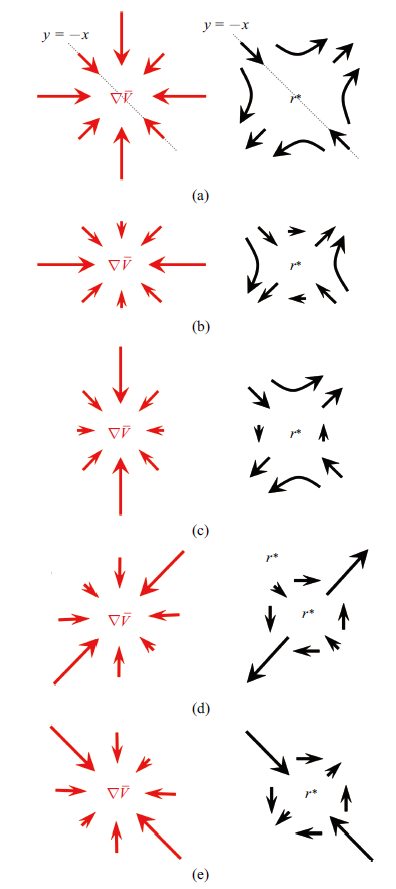Fig. 10   The relationship between $\nabla ^2\bar{{V}}$ and $r^\ast$. (a) Symmetry between $\nabla \bar{{V}}$ of soft region and quadrupolar nonaffine displacement. (b) Large gradient only in the $x$ direction. (c) Large gradient only in the $y$ direction. (d) Large gradient only in the $y = x$ direction. (e) Large gradient only in the $y = - x$ direction

### 2.5 基于原子体积拉普拉斯算子对STZ的预测

\begin{equation} \label{eq9} \mbox{Diff }\nabla \bar{{V}}=\sqrt {\left| {\bar{{V}}_x ^2-\bar{{V}}_y ^2} \right|} \end{equation}

\begin{equation} \label{eq10} \xi =\frac{-\nabla ^2\bar{{V}}\cdot \mbox{Diff }\nabla \bar{{V}}}{\varOmega _{\rm ave} } \end{equation}

\begin{equation} \label{eq11} S=\cup _{\gamma =0.1\% }^{9.0\% } S_\gamma \end{equation}

### 图11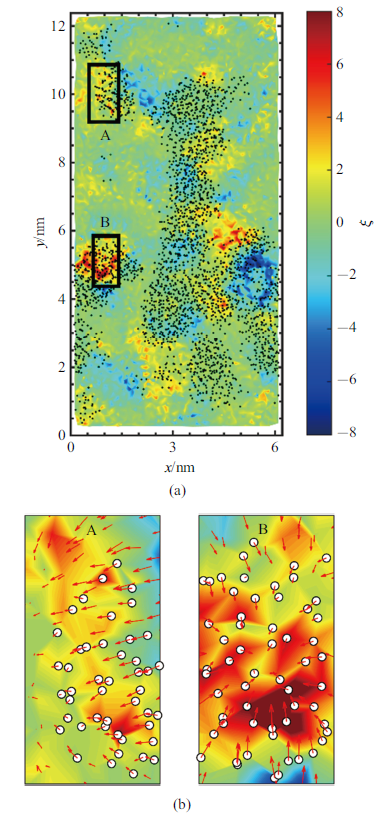Fig. 11   The contour plot of $\xi$, the spatial distribution of STZ atoms and $\nabla \bar{{V}}$

\begin{equation} \label{eq12} C(\gamma )=\frac{\hat{{S}}(\gamma )\cdot \hat{{P}}}{N_P }+\frac{\left[{I-\hat{{S}}(\gamma )} \right]\cdot \left[ {I-\hat{{P}}} \right]}{N-N_P}-1 \end{equation}

### 图12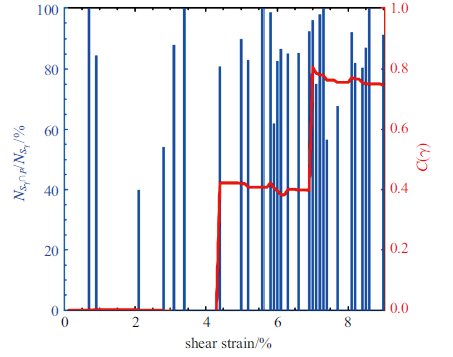Fig. 12   Accuracy in each step (bar plot) and cumulative correlation index (red solid curve)

Table 2  Averaged C(γ = 9.0%) of three sizes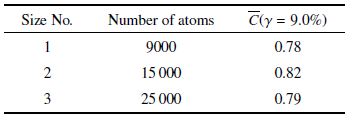## 参考文献 原文顺序 文献年度倒序 文中引用次数倒序 被引期刊影响因子

( Sun Yitao, Wang Chao, Yumiao , et al.

Recent progress of the glassy materials and physics

Acta Physica Sinica, 2018,67:126101 (in Chinese))

Wang Z, Wang WH .

Flow units as dynamic defects in metallic glassy materials

National Science Review, 2019,6:304-323

Wang N, Ding J, Yan F , et al.

Spatial correlation of elastic heterogeneity tunes the deformation behavior of metallic glasses

NPJ Computational Materials, 2018,4:19

Zhu F, Song S, Reddy KM , et al.

Spatial heterogeneity as the structure feature for structure-property relationship of metallic glasses

Nature Communications, 2018,9:3965

Qiao JC, Wang Q, Pelletier JM , et al.

Structural heterogeneities and mechanical behavior of amorphous alloys

Progress in Materials Science, 2019,104:250-329

Taylor GI .

The Mechanism of plastic deformation of crystals.

Part I. Theoretical. Proceedings of the Royal Society A: Mathematical, Physical and Engineering Sciences, 1934,145:362-387

Bernal JD .

A geometrical approach to the structure of liquids

Nature, 1959,183:141-147

Spaepen F .

A microscopic mechanism for steady state inhomogeneous flow in metallic glasses

Acta Metallurgica, 1977,25:407-415

( Wang Weihua .

The nature and properties of amorphous matter

Progress in Physics, 2013,33:177-351 (in Chinese))

Argon AS .

Plastic deformation in metallic glasses

Acta Metallurgica, 1979,27:47-58

Schall P, Weitz DA, Spaepen F .

Structural rearrangements that govern flow in colloidal glasses

Science, 2007,318:1895-1899

Sun BA, Wang WH .

The fracture of bulk metallic glasses

Progress in Materials Science, 2015,74:211-307

Maloney CE, Lemaître A .

Amorphous systems in athermal, quasistatic shear

Physical Review E, 2006,74:016118

Hu YC, Guan PF, Wang Q , et al.

Pressure effects on structure and dynamics of metallic glass-forming liquid

The Journal of Chemical Physics, 2017,146:024507

Wu YC, Wang B, Hu YC , et al.

The critical strain - A crossover from stochastic activation to percolation of flow units during stress relaxation in metallic glass

Scripta Materialia, 2017,134:75-79

Tian ZL, Wang YJ, Chen Y , et al.

Strain gradient drives shear banding in metallic glasses

Physical Review B, 2017,96:094103

Wei D, Yang J, Jiang MQ , et al.

Assessing the utility of structure in amorphous materials

The Journal of Chemical Physics, 2019,150:114502

Hu YC, Tanaka H, Wang WH .

Impact of spatial dimension on structural ordering in metallic glass

Physical Review E, 2017,96:022613

( Li Maozhi .

Five-fold local symmetries in metallic liquids and glasses

Acta Physica Sinica, 2017,66:176107 (in Chinese))

Zhang Q, Li QK, Zhao S , et al.

Structural characteristics in deformation mechanism transformation in nanoscale metallic glasses

Journal of Physics: Condensed Matter, 2019,31:455401

Fultz B .

Vibrational thermodynamics of materials

Progress in Materials Science, 2010,55:247-352

Chen K, Ellenbroek WG, Zhang Z , et al.

Low-Frequency Vibrations of Soft Colloidal Glasses

Physical Review Letters, 2010,105:025501

Yang J, Wang YJ, Ma E , et al.

Structural parameter of orientational order to predict the Boson vibrational anomaly in glasses

Physical Review Letters, 2019,122:015501

Baggioli M, Zaccone A .

Universal origin of boson peak vibrational anomalies in ordered crystals and in amorphous materials

Physical Review Letters. 2019,122:145501

Widmer-Cooper A, Perry H, Harrowell P , et al.

Irreversible reorganization in a supercooled liquid originates from localized soft modes

Nature Physics, 2008,4:711-715

Manning ML, Liu AJ .

Vibrational modes identify soft spots in a sheared disordered packing

Physical Review Letters, 2011,107:108302

Ding J, Patinet S, Falk ML , et al.

Soft spots and their structural signature in a metallic glass

Proceedings of the National Academy of Sciences, 2014,111:14052-14056

Rottler J, Schoenholz SS, Liu AJ .

Predicting plasticity with soft vibrational modes: From dislocations to glasses

PHYSICAL REVIEW E, 2014,89:42304

Ding J, Cheng YQ, Sheng H , et al.

Universal structural parameter to quantitatively predict metallic glass properties

Nature Communications, 2016,7:13733

Fan Z, Ding J, Li QJ , et al.

Correlating the properties of amorphous silicon with its flexibility volume

Physical Review B, 2017,95:144211

( Shi Beiji, He Guowei, Wang Shizhao .

Large-eddy simulation of flows with complex geometries by using the slip-wall model.

Lixue Xuebao/Chinese Journal of Theoretical and Applied Mechanics, 2019,51:754-766 (in Chinese))

( Meng Chunyu, Tang Zhengjun, Chen Mingxiang .

A large deformation elastoplastic model based on the intermediate configuration

Chinese Journal of Theoretical and Applied Mechanics, 2019,51(1):182-191 (in Chinese))

( Liu Jing, Li Jie, Zhang Heng .

Dimple's Vortex analysis based on eigenvalue of velocity gradient tensor

Chinese Journal of Theoretical and Applied Mechanics, 2019,51(3):826-834 (in Chinese))

Xu B, Falk ML, Li JF , et al.

Predicting shear transformation events in metallic glasses

Physical Review Letters, 2018,120:125503

DiDonna BA, Lubensky TC .

Nonaffine correlations in random elastic media

Physical Review E, 2005,72:066619

Cheng YQ, Ma E, Sheng HW .

Atomic level structure in multicomponent bulk metallic glass

Physical Review Letters, 2009, 102:

Plimpton S .

Fast parallel algorithms for short-range molecular dynamics

Journal of Computational Physics, 1995,117:1-19

Stukowski A .

Visualization and analysis of atomistic simulation data with OVITO-the Open Visualization Tool

Modelling and Simulation in Materials Science and Engineering, 2010,18:7

Wang B, Luo L, Guo E , et al.

Nanometer-scale gradient atomic packing structure surrounding soft spots in metallic glasses

npj Computational Materials, 2018,4:41

Maloney C, Lemaître A .

Universal breakdown of elasticity at the onset of material failure

Physical Review Letters, 2004,93:195501

Wang WH .

Correlation between relaxations and plastic deformation, and elastic model of flow in metallic glasses and glass-forming liquids

Journal of Applied Physics, 2011,110:053521

Falk ML, Langer JS .

Dynamics of viscoplastic deformation in amorphous solids

Physical Review E, 1998,57:7192-7205

Shimizu F, Ogata S, Li J .

Theory of Shear Banding in Metallic Glasses and Molecular Dynamics Calculations

Materials Transactions, 2007,48:2923-2927

Hu YC, Guan PF, Li MZ , et al.

Unveiling atomic-scale features of inherent heterogeneity in metallic glass by molecular dynamics simulations

Physical Review B, 2016,93:214202

Wei D, Yang J, Jiang MQ , et al.

Revisiting the structure-property relationship of metallic glasses: Common spatial correlation revealed as a hidden rule

Physical Review B, 2019,99:014115

Zink M, Samwer K, Johnson WL , et al.

Plastic deformation of metallic glasses: Size of shear transformation zones from molecular dynamics simulations

Physical Review B, 2006,73:172203

Zaccone A, Scossa-Romano E .

Approximate analytical description of the nonaffine response of amorphous solids

Physical Review B, 2011,83:184205

Şopu D, Stukowski A, Stoica M , et al.

Atomic-level processes of shear band nucleation in metallic glasses

Physical Review Letters, 2017,119:195503

Gendelman O, Jaiswal PK, Procaccia I , et al.

Shear transformation zones: State determined or protocol dependent?

EPL, 2015,109:16002

/

 〈〉